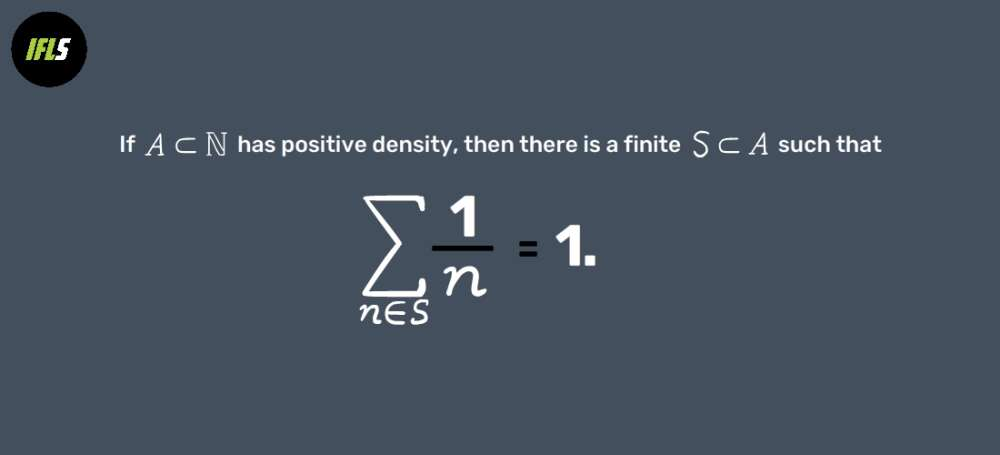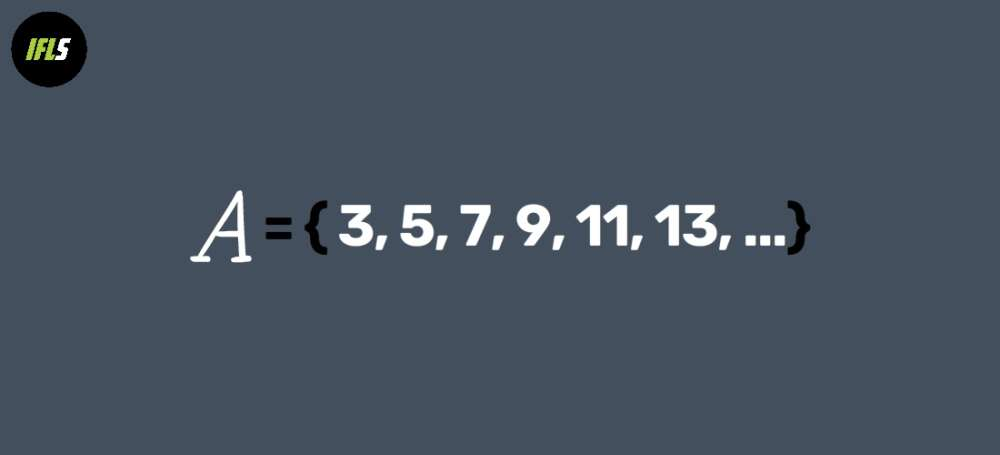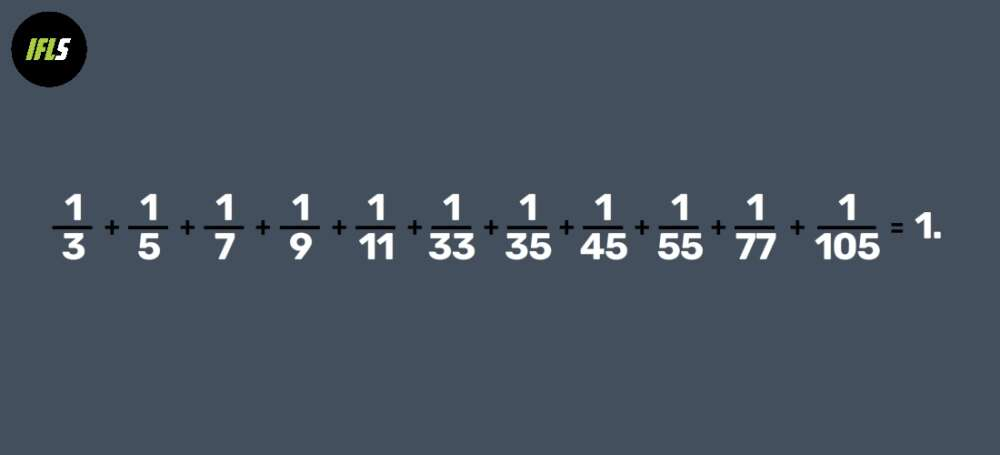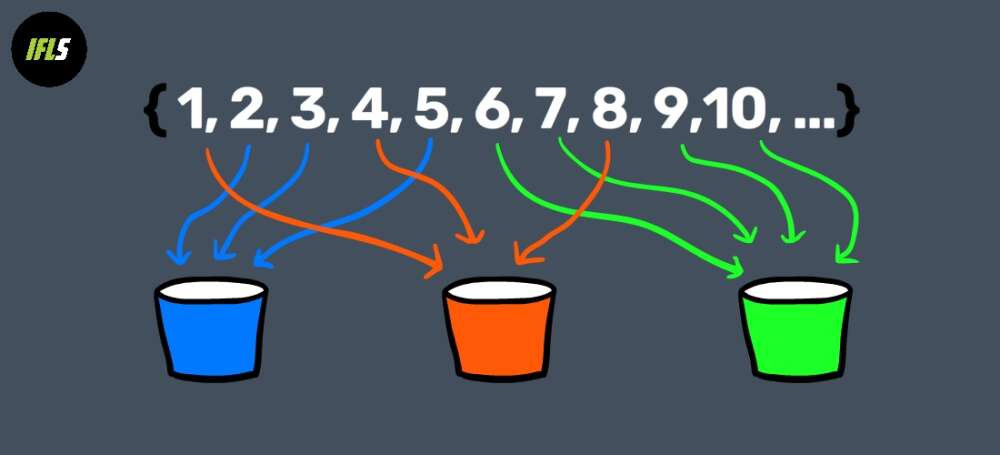TechnologyPUBLISHED

# Math Problem 3,500 Years In The Making Finally Gets A Solution24Comments390SharesThe problem has its roots in Ancient Egypt, but it was solved using cutting-edge mathematics. Image Credit: Zita/Peter Hermes Furian/Shutterstock, IFLScience

Here’s a question for all you math fans out there: if we give you a set of positive integers, can you pick out the ones whose reciprocals sum to one?

Let’s give an example: say we have the set {2, 4, 6, 8, 10, 12}. We can take out 2, 4, 6, and 12 from that set and we’ll find that

It’s not exactly an easy problem – but it doesn’t look too difficult either, right? However, it’s a version of this exact question that some mathematicians think “might be the oldest problem ever”. Now, thanks to a new paper by mathematician Thomas Bloom, it finally has an answer.

The density version of the Erd?s–Graham problem, to give its formal name, goes like this:Now, that’s basically math-speak for the question we asked at the top, but with one important difference: the set we start with is now infinitely large.

The good thing about math, though, is that finding infinitely large things is pretty easy. Here’s one: the set of all odd numbers bigger than two.This set doesn’t contain all positive integers, so it’s definitely a subset of the natural numbers – or in math terms,But it’s also a set with positive density. Now, that’s a fairly complex mathematical idea, but we can think of it like this: no matter how high we count, there’s a non-zero probability that we’re going to land on a number in the set A – in other words, an odd number bigger than one. Even if we’re up in the trillions, there’s still odd numbers, right?

So we’ve got our set A ? N with positive density; the next part of the problem tells us what we need to do with it. Just like before, the challenge is to find a group of numbers within the set which, when you take their reciprocal – that is, put them as the denominator in a fraction with numerator one – sum to one.Beautiful! But not enough: if we want to prove the statement, we need to be able to find those reciprocals in any set A that could possibly be chosen – and that is a much bigger problem.

“I just thought this was an impossible question that no one in their right mind could possibly ever do,” Andrew Granville, a mathematician from the University of Montreal, told Quanta Magazine. “I didn’t see any obvious tool that could attack it.”

As difficult as this problem was, it was almost by accident that Bloom found the solution. It began last September, when he was asked to present a paper on a similar – but less strong – result proved twenty years previously by a mathematician named Ernie Croot.

"I don't think I knew about this problem before I saw Croot's paper!" Bloom told IFLScience. "Certainly he wrote his paper long before I was taking any interest in mathematical matters."

What Croot had solved was known as the coloring version of the Erd?s–Graham problem. It’s called that because it involves “coloring” subsets – basically, you can think of it as separating up the set A by putting the elements into a finite number of different colored bins.

“Erd?s and I liked this problem so much that we posted a reward [of] \$500 for its solution,” Ronald Graham would later write.

“As it happened, Erd?s did not live to see the solution,” he added. “When I asked Ernie whether he would like a check for the \$500 signed by Erd?s, he said he would [be] pleased to be paid this way. (I kept a number of checks pre-signed by Erd?s for just such contingencies.)”Once the infinite set has been split into these colored bins, the coloring version of the Erd?s–Graham problem then says that we can definitely find one bin which contains numbers whose reciprocals add to one. It was unsolved for around twenty years before Croot eventually cracked it, and his much-celebrated proof was published in the Annals of Mathematics in 2003.

“Croot’s argument is a joy to read,” mathematician Giorgis Petridis from the University of Georgia told Quanta. “It requires creativity, ingenuity and a lot of technical strength.”

The coloring problem is very similar to the density problem – both require us to find a subset of numbers whose reciprocals sum to one – but it’s different in one very important way.

In the coloring problem, the entire set A has been split into bins. You don’t know exactly how it’s been split up, but that doesn’t really matter – all you need to show is that there’s one bin with numbers nice enough to work for the sum. That’s exactly what Croot did in his paper: he constructed a proof to show that at least one bin will always have enough of these nice numbers – numbers with low prime factors, or in mathematical jargon, “smooth numbers” – to satisfy the theorem.

But with the density version of the problem, that shortcut isn’t available. You can’t just choose the most convenient bin – you might have been given a subset S with some really horrible numbers.

"It was something I couldn’t quite get around,” Croot told Quanta.

But while he was reading up on the paper, Bloom realized something – the coloring problem and the density problem were really one and the same.

"It's of course hard to think about hypotheticals, but I'm quite sure that without seeing the method of Croot, I wouldn't have had an idea about where to start," Bloom told IFLScience. "Croot's method really is extraordinary, and he deserves 99% of the credit -- all I did was just push a little further on a door he had already opened."

When Croot proved that one bin contained a set of smooth enough numbers to satisfy the theorem, Bloom noticed, he had really just proved a special case of the density problem. That meant that with a little work, Bloom could use that proof to finish the density problem – all he had to do was show that the result would be the same even if those numbers were made a little less smooth, and the density problem would be completely solved.

"Roughly speaking, the idea is to prove the existence of a solution by counting solutions, which we do using an exponential sum," Bloom explained. "That exponential sum can be broken into two pieces: a 'major arc contribution' (which we can calculate explicitly, and is large) and a 'minor arc contribution' (which we have no idea how to calculate explicitly, but which we can show is small)."

Croot's "real genius," Bloom told IFLS, was to figure out a new way of thinking about the minor arc contribution – he "turned [it] into a different kind of question," Bloom said. Instead of trying to calculate the value, he looked at how multiples in the set are distributed along the number line.

"Provided there are lots of 'gaps' between these multiples, the minor arc contribution is small," explained Bloom. "Croot then used information about the primes dividing numbers of the given set to show that there must be lots of gaps as required."

So much for the coloring version of the problem – now Bloom needed to make it work for the density version. Luckily, though, much of the work had already been done for him – the methods Bloom used were really “a stronger form of the ideas introduced by Croot,” his paper explains.

"Croot's method works for any set which is large and has no large prime divisors. The last part is what we need to remove to get the density version," Bloom told IFLS. "To do this, I improved on Croot's method roughly by working 'locally' for each large prime – so instead of showing there are lots of gaps between multiples of the whole set, just looking at gaps between multiples of those numbers in the set divisible by each large prime, considered one at a time, rather than separately."

He also made his life easier by finding sums not to one, but smaller fractions: “You’re not finding 1 honestly,” he told Quanta. “You’re finding maybe 1/3, but if you do that three times in three different ways, then just add them to each other and you’ve got 1.”

With his new proof, Bloom has solved a question with roots all the way back in Ancient Egypt – but if you think this is the end for the problem of sets and sums, you clearly haven’t met many number theorists. Bloom's next step is set to take this ancient problem straight into the modern age: "I'm currently working on formalising the proof in Lean, which is what's known as a 'proof assistant'," he told IFLS.

"This is an exciting new area, where we can have computers formally check proofs to a much greater degree of rigour than is usually the case in human maths," he said.

“[Bloom’s proof is] an outstanding result,” University of British Columbia mathematician Izabella ?aba told Quanta. “Combinatorial and analytic number theory has evolved a lot over the last 20 years. That made it possible to come back to an old problem with a new perspective and with more efficient ways to do things.”

### ARTICLE POSTED INTechnology
•• mathematics